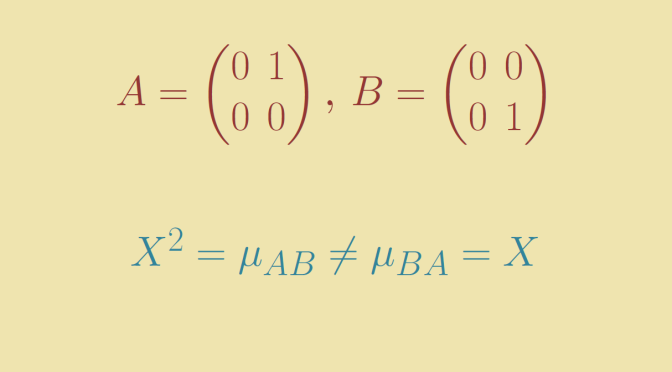# Two matrices A and B for which AB and BA have different minimal polynomials

We consider here the algebra of matrices $$\mathcal{M}_n(\mathbb F)$$ of dimension $$n \ge 1$$ over a field $$\mathbb F$$.

It is well known that for $$A,B \in \mathcal{M}_n(\mathbb F)$$, the characteristic polynomial $$p_{AB}$$ of the product $$AB$$ is equal to the one (namely $$p_{BA}$$) of the product of $$BA$$. What about the minimal polynomial?

Unlikely for the characteristic polynomials, the minimal polynomial $$\mu_{AB}$$ of $$AB$$ maybe different to the one of $$BA$$.

Consider the two matrices $A=\begin{pmatrix} 0 & 1\\ 0 & 0\end{pmatrix} \text{, } B=\begin{pmatrix} 0 & 0\\ 0 & 1\end{pmatrix}$ which can be defined whatever the field we consider: $$\mathbb R, \mathbb C$$ or even a field of finite characteristic.

One can verify that $AB=A=\begin{pmatrix} 0 & 1\\ 0 & 0\end{pmatrix} \text{, } BA=\begin{pmatrix} 0 & 0\\ 0 & 0\end{pmatrix}$

As $$BA$$ is the zero matrix, its minimal polynomial is $$\mu_{BA}=X$$. Regarding the one of $$AB$$, we have $$(AB)^2=A^2=0$$ hence $$\mu_{AB}$$ divides $$X^2$$. Moreover $$\mu_{AB}$$ cannot be equal to $$X$$ as $$AB \neq 0$$. Finally $$\mu_{AB}=X^2$$ and we verify that $X^2=\mu_{AB} \neq \mu_{BA}=X.$